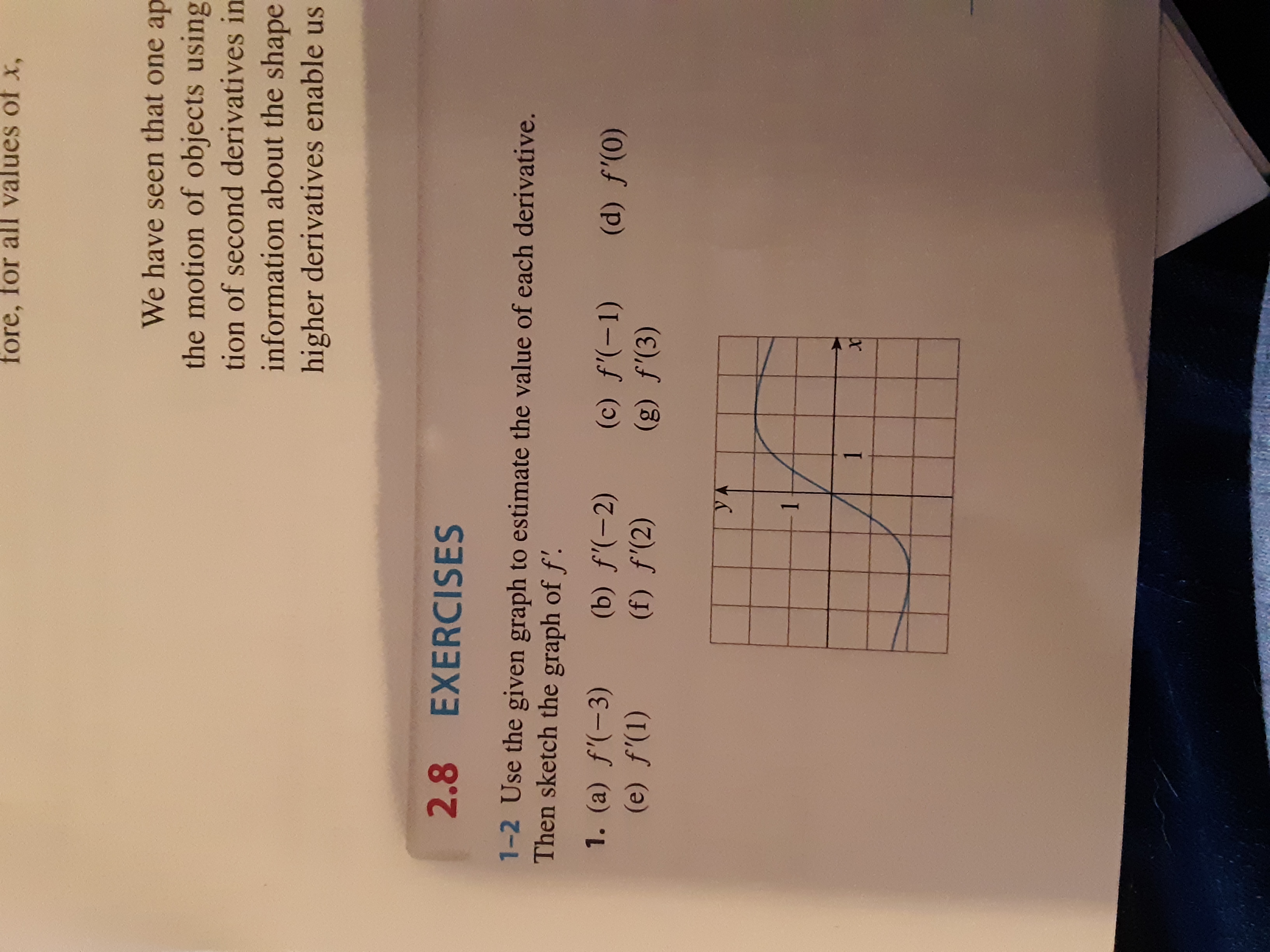# fore, for all values of X,We have seen that one apthe motion of objects usingtion of second derivatives ininformation about the shapehigher derivatives enable us2.8EXERCISES1-2 Use the given graph to estimate the value of each derivative.Then sketch the graph of f'.1. (a) f'(-3)(b) f'(-2)(f) f'(2)(c) f'(-1)(g) f'(3)(d) f'(0)(e) f'(1)YA11

Question
1 views

See image below.help_outlineImage Transcriptionclosefore, for all values of X, We have seen that one ap the motion of objects using tion of second derivatives in information about the shape higher derivatives enable us 2.8 EXERCISES 1-2 Use the given graph to estimate the value of each derivative. Then sketch the graph of f'. 1. (a) f'(-3) (b) f'(-2) (f) f'(2) (c) f'(-1) (g) f'(3) (d) f'(0) (e) f'(1) YA 1 1 fullscreen
check_circle

Step 1

Since we only answer up to 3 sub-parts, we’ll answer the first 3. Please resubmit the question and specify the other subparts (up to 3) you’d like answered.

f ' (x) means slope of f(x) at x.

a) f'(-3) is slope of f(x) at x=-3.

We will consider the line joining (-2,-2) and (-4, -5/3)

[ Got 5/3 using 1 + 2/3 , because the upper part of the box looks like 2/3 units]

Step 2

Then we find the slope between (-2,-2) and (-4,-5/3)

Step 3

b) There is a local minimum at x=-2, this means x=2 is a critic...

### Want to see the full answer?

See Solution

#### Want to see this answer and more?

Solutions are written by subject experts who are available 24/7. Questions are typically answered within 1 hour.*

See Solution
*Response times may vary by subject and question.
Tagged in

### Calculus